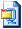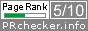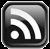# LEARN MS-ACCESS TIPS AND TRICKS

Learn Microsoft Access Advanced Programming Techniques, Tips and Tricks.

### Introduction.

If you have landed straight on this page then please refer to my earlier Article: Command-Button Animation to link the essential library files to your project.  The list of library files and guidelines for attaching them to your project are given there. These files are required to run the program on this page successfully.

The function given below creates an interesting and attractive heading style like the Sample image showed above. The font, font-style (Bold, Italic), shadow, border, and fore-color can be customized, after creating the sample heading on a new form.

Copy and Paste the VBA Code given below in a new global module in your database. If you have already copied the first three functions (function names given below) and the global declarations from the earlier Post: Create 3D Headings on Forms then copy and paste the last function Border3D() only.

The following common functions are used by the main program Border3D() for all other 2D/3D styles:

• FormTxtLabels()
• Validate_Dup()
• MsgLabel()

### The Functions VBA Code.

If you have already copied them earlier then copy only the last Function: Border3D()

```Option Compare Database
Option Explicit
'-- Global declarations
Private Const lngheight as long = 0.45 * 1440
Private Const lngWidth as long = 4.5 * 1440
Private Const intFontSize as integer = 26
Private Const intTextAlign as integer = 0
Private Const intBackStyle as integer = 0
Private Const LngI as long = 0.0104 * 1440
Private Const intX as long = 0.15 * 1440
Private Const intY as long = 0.15 * 1440
Dim MyFrm As Form

Public Function FormTxtLabels(Optional ByVal ControlType As Integer) As String
'---------------------------------------------------
'Author : a.p.r. pillai
'Date : September 2006
'ControlType = 0 for label
'ControlTYPE = 1 for TextBox
'---------------------------------------------------
Dim ctl As Control
'On Error GoTo FormTxtLabels_Err
If ControlType > 0 Then ControlType = 1

Set MyFrm = CreateForm

If ControlType = 1 Then
Set ctl = CreateControl(MyFrm.NAME, acTextBox, acDetail, , , (0.2 * 1440), (0.2 * 1440), lngWidth, lngheight)

With ctl
.ControlSource = "=" & Chr(34) & "msaccesstips.com" & Chr(34)
End With
Else
Set ctl = CreateControl(MyFrm.NAME, acLabel, _acDetail, , , (0.2 * 1440), (0.2 * 1440), lngWidth, lngheight)

With ctl
.Caption = "msaccesstips.com"
End With

End If

FormTxtLabels = MyFrm.NAME

FormTxtLabels_Exit:
Exit Function
```

```Public Function Validate_Dup(ByRef MyFrm As Form, ByVal intNooflabels As Integer) As Integer
'---------------------------------------------------
'Author : a.p.r. pillai
'Date : September 2006
'ControlType = 0 for label
'ControlTYPE = 1 for TextBox
'---------------------------------------------------
Dim mysec As Section, lblcount As Integer
Dim myctrl As Control, newctrl As Control, j As Integer
Dim lngx As Long, lngY As Long, lngH As Long, lngW As Long
Dim strCap As String, ctrltype As Integer, intlbls As Integer
Dim ctrlName() As String, ctrlIndex() As Integer, i As Long
Dim strFont As String, intFntSize As Integer, x As Integer
Dim intFntWeight As Integer

'On Error GoTo Validate_Dup_Err

Set mysec = MyFrm.Section(acDetail)
intlbls = mysec.Controls.Count - 1

Set myctrl = mysec.Controls(0)
ctrltype = myctrl.ControlType
intNooflabels = intNooflabels - 1
If intlbls > 0 Then
ReDim ctrlName(intlbls) As String
ReDim ctrlIndex(intlbls) As Integer
End If
If ctrltype = 109 And intlbls > 0 Then
For j = 0 To intlbls
Set myctrl = mysec.Controls(j)
ctrlIndex(j) = myctrl.ControlType
ctrlName(j) = myctrl.NAME
Next
For j = 0 To intlbls
If ctrlIndex(j) = 100 Then
DeleteControl MyFrm.NAME, ctrlName(j)
End If
Next
intlbls = mysec.Controls.Count - 1
End If

Set myctrl = mysec.Controls(0)
If intlbls < intNooflabels Then
With myctrl
lngx = .Left
lngY = .Top
lngW = .Width
lngH = 0.0208 * 1440 ' 0.0208 inches
strFont = .FontName
intFntSize = .FontSize
intFntWeight = .FontWeight
End With

If ctrltype = 100 Then
strCap = myctrl.Caption
ElseIf ctrltype = 109 Then
strCap = myctrl.ControlSource
End If
If ctrltype = 109 And intlbls > 0 Then
For j = 0 To intlbls
Set myctrl = mysec.Controls(j)
ctrlIndex(j) = myctrl.ControlType
ctrlName(j) = myctrl.NAME
Next
For j = 0 To intlbls
If ctrlIndex(j) = 100 Then
DeleteControl MyFrm.NAME, ctrlName(j)
End If
Next
intlbls = mysec.Controls.Count - 1
Set myctrl = mysec.Controls(intlbls)
With myctrl
lngx = .Left
lngY = .Top
lngW = .Width
lngH = .Height
ctrltype = .ControlType
strFont = .FontName
intFntSize = .FontSize
intFntWeight = .FontWeight
End With
End If

i = 0.0104 * 1440 + lngH ' 0.0104 inches
lngY = lngY + i
For j = intlbls + 1 To intNooflabels

Set newctrl = CreateControl(MyFrm.NAME, ctrltype, _acDetail, "","", lngx, lngY, lngW, lngH)

If ctrltype = 100 Then
newctrl.Caption = strCap
newctrl.FontName = strFont
newctrl.FontSize = intFntSize
newctrl.FontWeight = intFntWeight
Else
newctrl.ControlSource = strCap
newctrl.FontName = strFont
newctrl.FontSize = intFntSize
newctrl.FontWeight = intFntWeight
End If
lngY = lngY + i
Next
End If

If intlbls > intNooflabels Then
For j = intNooflabels + 1 To intlbls
Set myctrl = mysec.Controls(j)
ctrlIndex(j) = myctrl.ControlType
ctrlName(j) = myctrl.NAME
Next
For j = intNooflabels + 1 To intlbls
DeleteControl MyFrm.NAME, ctrlName(j)
Next
Validate_Dup = 0
End If

intlbls = mysec.Controls.Count - 1
Set myctrl = mysec.Controls(0)
ctrltype = myctrl.ControlType
If ctrltype = 109 Then
For j = 0 To intlbls
Set myctrl = mysec.Controls(j)
With myctrl
.Enabled = False
.Locked = True
.SpecialEffect = 0
End With
Next
End If
Validate_Dup = 0

Validate_Dup_Exit:
Exit Function

Validate_Dup_Err:
MsgBox Err.Description, ,"Validate_Dup"
Validate_Dup = 1
Resume Validate_Dup_Exit
End Function
```

```Public Function MsgLabel()
'------------------------------------------------------------
'Author : a.p.r. pillai
'Date : September 2006
'------------------------------------------------------------
Dim mySection As Section, ctl As Control, xForm As Form
Dim l As Long, t As Long, w As Long, h As Long, F As Long
F = 1440
l = 0.5 * F: t = 1.2 * F: w = 3.6563 * F: h = 0.4896 * F 'values in inches
On Error Resume Next
Set mySection = MyFrm.Section(acDetail)
Set ctl = CreateControl(MyFrm.NAME, acLabel, _acDetail, , "", l, t, w, h)
ctl.Caption = "Click outside the Controls and Drag Over. "  & "Display the Property Sheet. " & "Type New Text for Caption/Control " & "Source Property area for Label/ Text Boxes. " & "Copy and Paste the Controls to " & "Target Form/Report Area."
End Function
```

```Public Function Border3D(ByVal intStyle As Integer, ByVal intForeColor As Integer, _
ByVal intBorderColor As Integer, Optional ByVal Label0Text1 As Integer) As String
'--------------------------------------------------
'Author : a.p.r. Pillai
'Date   : September 2006
'--------------------------------------------------
Dim intlbls As Integer
Dim j As Integer, ForeColor As Long, BorderColor As Long
Dim lblName() As String, X As Integer, mySection As Section
Dim l As Long, t As Long, I As Long, intFSize As Integer
On Error Resume Next
I = 0.0104 * 1440 ' 0.0104 inches
Border3D = FormTxtLabels(Label0Text1)
Set mySection = MyFrm.Section(acDetail)
intlbls = mySection.Controls.Count - 1
On Error GoTo Border3D_Err
X = Validate_Dup(MyFrm, 7) ' check type and duplicate
If X = 1 Then
Exit Function
End If
intlbls = mySection.Controls.Count - 1
X = intForeColor
intForeColor = IIf(X < 0, 0, IIf(X > 15, 15, intForeColor))
X = intBorderColor
intBorderColor = IIf(X < 0, 0, IIf(X > 15, 15, intBorderColor))
X = intStyle
intStyle = IIf(X < 0, 0, IIf(X > 3, 3, intStyle))
ReDim lblName(0 To intlbls) As String
ForeColor = QBColor(intForeColor)
BorderColor = QBColor(intBorderColor)
For j = 0 To intlbls
lblName(j) = mySection.Controls(j).NAME
Next
For j = 0 To intlbls
With mySection.Controls(lblName(j))
.Height = lngheight
.Width = lngWidth
.FontName = "Times New Roman"
intFSize = .FontSize
If intFSize < intFontSize Then
.FontSize = intFontSize
End If
.FontUnderline = False
.TextAlign = intTextAlign
.BackStyle = intBackStyle
End With
Next
mySection.Controls(lblName(intlbls)).ForeColor = ForeColor
For j = 0 To intlbls - 1
mySection.Controls(lblName(j)).ForeColor = BorderColor
Next
l = intX: t = intY
With mySection.Controls(lblName(intlbls))
.Left = l
.Top = t
End With
For j = 2 To intlbls - 1
With mySection.Controls(lblName(j))
Select Case j
Case 2
.Left = l + I
.Top = t + I
Case 3
.Left = l + I
.Top = t - I
Case 4
.Left = l - I
.Top = t + I
Case 5
.Left = l - I
.Top = t - I
End Select
End With
Next
For j = 0 To 1
With mySection.Controls(lblName(j))
'.ForeColor = 9868950
.ForeColor = 0
If j = 0 Then
Select Case intStyle
Case 0
.Left = l - (I * 3)
.Top = t - (I * 3)
Case 1
.Left = l - (I * 3)
.Top = t + (I * 3)
Case 2
.Left = l + (I * 3)
.Top = t - (I * 3)
Case 3
.Left = l + (I * 3)
.Top = t + (I * 3)
End Select
Else
Select Case intStyle
Case 0
.Left = l - (I * 2)
.Top = t - (I * 2)
Case 1
.Left = l - (I * 2)
.Top = t + (I * 2)
Case 2
.Left = l + (I * 2)
.Top = t - (I * 2)
Case 3
.Left = l + (I * 2)
.Top = t + (I * 2)
End Select
End If
End With
Next
MsgLabel
Border3D_Exit:
Exit Function

Border3D_Err:
Msgbox Err.Description,, "Border3D"
Resume Border3D_Exit
End Function
```

### How to Create

To create the Border3D Heading Text, press ALT+F11 to open VBA Window and press Ctrl+G to display the Debug Window (Immediate Window). Type the following line in the immediate window and press the Enter key:

Border3D 1,4,15,0

The module window will flash for a moment as if it is refreshed. Minimize the VBA Window and you will see a new form created and kept minimized on the taskbar by the program. Restore the form and save it with the Heading Text.

First, let us get familiarized with the Parameter values to the function. The first three Parameters are mandatory.   If any of them is omitted then the program will show Error Message 'Parameter not optional' and the fourth value is optional.

### Parameter Values

The first parameter value represents the shadow position. The value range and their shadow positions are as given below:

• 0 - Top Left
• 1 - Bottom Left
• 2 - Top Right
• 3 - Bottom Right

By using one of the four values as the first parameter you can display the shadow tilted to any one of the four corners of the heading.

The second parameter value 4 (Red Color) is Text color and the value range is 0 to 15.  You can find the color numbers and their description here.

The third parameter value 15 draws white-colored borders to the text and the value range is 0 to 15.

The fourth parameter value is optional, if omitted 0 is assumed and it will create a Label based Heading Text, 1 will create a Text Box based Heading.

### Customizing Text

After creating the 3D Heading, to select all the labels together, click and hold the left mouse button, somewhere outside near the labels and drag the mouse over them. After selecting all the labels together display the property sheet (Press F4 or View--> Property) and change the Caption property value with your own required text.  Change the font, font size, font style bold, or italic to your liking.

To de-select, all the labels, click somewhere on the form away from the labels. Now, you can change the fore-color of the heading to your choice.  Click on the top layer of the labels carefully so that you don’t disturb the arrangement of labels, and change the fore-color of the Heading to your liking.  Now, select all the labels together, as you did earlier, press Ctrl+C to copy them into the clipboard. Open your target Form or Report in design view and paste the heading on it. This form you can save as a Template so that you can copy, modify, and use it on other Forms or Reports without running the program from the module window again.Download Demo Database
Share:

Comments subject to moderation before publishing.

## TranslateSubscribe in a readerYour email address:

Delivered by FeedBurner